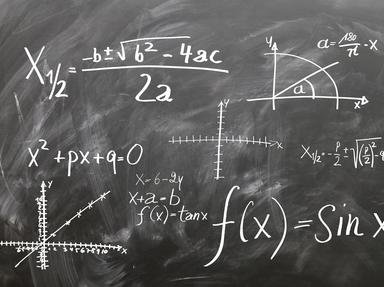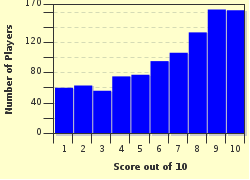# I Am Special! Trivia Quiz

### Every number is special. Ten numbers will give a brief introduction about themselves and you have to reveal their identities. Enjoy!

A multiple-choice quiz by Matthew_07. Estimated time: 8 mins.

Author
Matthew_07
Time
8 mins
Type
Multiple Choice
Quiz #
291,027
Updated
Dec 31 21
# Qns
10
Difficulty
Tough
Avg Score
6 / 10
Plays
5236
Awards
Top 5% quiz!
Last 3 plays: mazza47 (10/10), GuyCognito (7/10), calmdecember (2/10).
This quiz has 2 formats: you can play it as a or as shown below.
Scroll down to the bottom for the answer key.
1. There are only two numbers that are twice the sum of their individual digits. One of them is 0 and I am the other one. I am a multiple of 9. Which number am I?

#### NEXT>

2. You may have heard of the famous Fibonacci numbers. (1, 1, 2, 3, 5... where the next number is the sum of its previous two numbers) Well, I am one of them. What makes me more special is that I am the largest cube in this sequence. Which number am I?

#### NEXT>

3. No other numbers are more special than me. I am the only number that is located between a square and a cube. More hints for you; I am smaller than 50 and I am an even number. Which number am I?

#### NEXT>

4. I know number 10 is special, because we use it in our number system. However, I am not talking about number 10. I am the smallest number with 10 divisors. More clues to help you; I am smaller than 50 and a multiple of 12. Which number am I?

#### NEXT>

5. A bit of thinking here for this question. I am the largest four-digit number with different digits, each greater than the one before it. Which number am I?

#### NEXT>

6. I am the age of the oldest person in the Bible - Methuselah when he died. I am a three-digit odd palindromic number that ends in 9. The sum of my individual digits is 24. Which number am I?

#### NEXT>

7. If you have a calculator with you now, you can find me. I am the answer for 1^7 + 2^6 + 3^5 + 4^4 + 5^3 + 6^2 + 7^1. But if you do not have one, never mind. I end in 2 and the sum of my individual digits is 12. I am greater than 700 but smaller than 800. Which number am I?

#### NEXT>

8. Another palindromic number for you. I am the smallest five-digit palindromic number that ends in 9. Which number am I?

#### NEXT>

9. If you are obsessed with Fibonacci numbers (1, 1, 2, 3, 5...) then this is the question for you. I am the largest square in the Fibonacci sequence. I am smaller than 150 and a multiple of 12. Which number am I?

#### NEXT>

10. You may think I am a joke, but I am the smallest whole number with three different digits. Which number am I?

 (Optional) Create a Free FunTrivia ID to save the points you are about to earn:Select a User ID:Choose a Password:Your Email:

Quiz Answer Key and Fun Facts
1. There are only two numbers that are twice the sum of their individual digits. One of them is 0 and I am the other one. I am a multiple of 9. Which number am I?

Since the number is a two-digit number and it is a multiple of 9, it could be 18, 27, 45... So let's consider 18 first. Since 1 + 8 = 9 x 2 = 18, 18 is the answer we are looking for.
2. You may have heard of the famous Fibonacci numbers. (1, 1, 2, 3, 5... where the next number is the sum of its previous two numbers) Well, I am one of them. What makes me more special is that I am the largest cube in this sequence. Which number am I?

In fact, there are only two cubes in the whole Fibonacci sequence, namely 1 and 8.
3. No other numbers are more special than me. I am the only number that is located between a square and a cube. More hints for you; I am smaller than 50 and I am an even number. Which number am I?

First, we list out all the squares and cubes smaller than 50 and arrange them in ascending order. So we have 1, 4, 8, 9, 16, 25, 27, 36, 49. After coming up with this list, it could be easily seen that 26 is the number that fits the description.
4. I know number 10 is special, because we use it in our number system. However, I am not talking about number 10. I am the smallest number with 10 divisors. More clues to help you; I am smaller than 50 and a multiple of 12. Which number am I?

Since the number is a multiple of 12, it could be 12, 24, 36 and 48.
Divisors of 12: 1, 2, 3, 4, 6, 12. (6 divisors)
Divisors of 24: 1, 2, 3, 4, 6, 8, 12, 24. (8 divisors)
Divisors of 36: 1, 2, 3, 4, 6, 9, 12, 18, 36. (9 divisors)
Divisors of 48: 1, 2, 3, 4, 6, 8, 12, 16, 24, 48. (10 divisors)
5. A bit of thinking here for this question. I am the largest four-digit number with different digits, each greater than the one before it. Which number am I?

Our choices can be narrowed down to 1234, 2345, 3456, 4567, 5678 and 6789. Clearly, 6789 is the answer for this question.
6. I am the age of the oldest person in the Bible - Methuselah when he died. I am a three-digit odd palindromic number that ends in 9. The sum of my individual digits is 24. Which number am I?

The number is in the form of 9_9. So we will just have to fill in the blank. 24 - 9 - 9 = 6. So 969 is the number we are looking for.
7. If you have a calculator with you now, you can find me. I am the answer for 1^7 + 2^6 + 3^5 + 4^4 + 5^3 + 6^2 + 7^1. But if you do not have one, never mind. I end in 2 and the sum of my individual digits is 12. I am greater than 700 but smaller than 800. Which number am I?

The number is in the form of _ _ 2. Since the sum of its individual digits is 12, this leaves us with 732.
8. Another palindromic number for you. I am the smallest five-digit palindromic number that ends in 9. Which number am I?

The number is in the form of 9_ _ _ 9. Since the question asks for the smallest number, 90009 is the answer.
9. If you are obsessed with Fibonacci numbers (1, 1, 2, 3, 5...) then this is the question for you. I am the largest square in the Fibonacci sequence. I am smaller than 150 and a multiple of 12. Which number am I?

We are given the hints that the number is smaller than 150 but greater than 100. Besides, it is a multiple of 12. This leaves us with 108, 120, 132 and 144. Out of these 4 numbers, only 144 is a square number and it is a Fibonacci number. 1, 1, 2, 3, 5, 8, 13, 21, 34, 55, 89, 144. It is the twelfth Fibonacci number.
10. You may think I am a joke, but I am the smallest whole number with three different digits. Which number am I?

This question just requires some logical thinking. The first few 3-digit numbers are 100, 101, 102, 103... 102 is the number we are looking for.
Source: Author Matthew_07

This quiz was reviewed by FunTrivia editor crisw before going online.
Any errors found in FunTrivia content are routinely corrected through our feedback system.
Most Recent Scores
Jan 26 2023 : mazza47: 10/10
Jan 19 2023 : GuyCognito: 7/10
Jan 02 2023 : calmdecember: 2/10
Dec 31 2022 : Guest 73: 10/10
Dec 22 2022 : Guest 147: 10/10
Dec 14 2022 : Guest 166: 5/10

Score DistributionRelated Quizzes
This quiz is part of series Guess the Numbers:

Guess the numbers (positive integers) based on the clues and descriptions given.

1. I Am Special! Tough
2. I Am Special, Too! Tough
3. Numbers Costume Party! Average
4. Palindromic Numbers Party! Average
5. Mathematicians and Discoveries! Average
6. Dealing with Dark Digits Average
7. Sorry, Wrong Number Easier